# 線性代數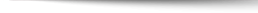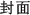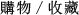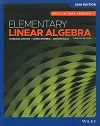## Elementary Linear Algebra 12/e Asia Edition

+作者：

### Anton

+年份：
2019 年12 版
+ISBN：
9781119666257
+書號：
MA0472PC
+規格：

+頁數：
756
+出版商：
John Wiley

\$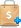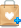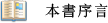• New Application Section - A new section on the mathematics of facial recognition has been added to Chapter 10.
• Earlier Linear Transformations - Selected material on linear transformations that was covered later in the previous edition has been moved to Chapter 1 to provide a more complete early introduction to the topic. Specifically, some of the material in Sections 4.10 and 4.11 of the previous edition was extracted to form the new Section 1.9, and the remaining material is now in Section 8.6.
• New Section 4.3 Devoted to Spanning Sets - Section 4.2 of the previous edition dealt with both subspaces and spanning sets. Classroom experience has suggested that too many concepts were being introduced at once, so we have slowed down the pace and split off the material on spanning sets to create a new Section 4.3.
• New Examples - New examples have been added, where needed, to support the exercise sets.
• New Exercises - New exercises have been added with special attention to the expanded early introduction to linear transformations.• Interrelationships Among Concepts - One of our main pedagogical goals is to convey to the student that linear algebra is not a collection of isolated definitions and techniques, but is rather a cohesive subject with interrelated ideas. One way in which we do this is by using a crescendo of theorems labeled "Equivalent Statements" that continually revisit relationships among systems of equations, matrices, determinants, vectors, linear transformations, and eigenvalues. To get a general sense of this pedagogical technique see Theorems 1.5.3, 1.6.4, 2.3.8, 4.9.8, 5.1.5, 6.4.5, and 8.2.4.
• Smooth Transition to Abstraction - Because the transition from Euclidean spaces to general vector spaces is difficult for many students, considerable effort is devoted to explaining the purpose of abstraction and helping the student .to "visualize" abstract ideas by drawing analogies to familiar geometric ideas.
• Mathematical Precision - We try to be as mathematically precise as is reasonable for students at this level. But we recognize that mathematical precision is something to be learned, so proofs are presented in a patient style that is tailored for beginners:
• Suitability for a Diverse Audience - The text is designed to serve the needs of students in engineering, computer science, biology, physics, business, and economics, as well as those majoring in mathematics.
• Historical Notes - We feel that it is important to give students a sense of mathematical history and to convey that real people created the mathematical theorems and equations they are studying. Accordingly, we have included numerous "Historical Notes" that put various topics in historical perspective.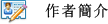Howard Anton - Professor Emeritus, Drexel University
Chris Rorres - Professor Emeritus, Drexel University
Anton Kaul - Professor, California Polytechnic State University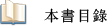1 Systems of Linear Equations and Matrices
2 Determinants
3 Euclidean Vector Spaces
4 General Vector Spaces
5 Eigenvalues and Eigenvectors
6 Inner Product Spaces
8 General Linear Transformations
9 Numerical Methods
10 Applications of Linear Algebra
Supplemental Online Topics
• Linear Programming - A Geometric Approach
• Linear Programming - Basic Concepts
• Linear Programming - The Simplex Method
• Vectors in Plane Geometry
• Equilibrium of Rigid Bodies
• The Assignment Problem
• The Determinant Function
• Leontief Economic Models
Appendix A Working with Proofs
Appendix B Complex Numbers